2020-12-29 21:26:31 +0200 received badge ● Popular Question (source) 2020-12-29 21:26:31 +0200 received badge ● Notable Question (source) 2020-10-09 05:26:58 +0200 received badge ● Famous Question (source) 2020-09-22 05:16:29 +0200 received badge ● Popular Question (source) 2020-02-20 02:06:06 +0200 marked best answer Partially commutative monoid of a graph I am interested in the free partially commutative monoid associated to a graph whose definition can be seen here www.sciencedirect.com/science/article... and here https://en.m.wikipedia.org/wiki/Trace.... Basically I need a free monoid in which some variables commutes. My questions are whether this notion is already implemented in Sage? If not how to implement this in Sage? 2020-02-20 02:06:00 +0200 received badge ● Popular Question (source) 2020-01-10 07:16:56 +0200 received badge ● Notable Question (source) 2019-09-10 11:51:05 +0200 received badge ● Associate Editor (source) 2019-09-10 11:41:54 +0200 asked a question $p$-adic extension of $n$th root of unity. I have used the following command to define the 5-adic Unramified extension ring in c defined by the polynomial $x^3 + 3x + 3$: Sage: R. = zq(125, prec=20)  Now, I want to find all the $n$th root of unity in this ring for $n$ dividing $124$. I dont know, how the $n$-th roots are implemented. Kindly help me with this. Thank you. 2019-09-10 11:36:10 +0200 received badge ● Enthusiast 2019-08-27 10:59:52 +0200 received badge ● Nice Question (source) 2019-08-19 12:05:49 +0200 asked a question Automorphism group of Coxeter groups Coxeter groups can be invoked using the comment W = CoxeterGroup(['A',3],implementation='reflection')  Now, W has three simple reflections S_1, S_2 and S_3. and I am interested in studying the automorphism S_1 -----> S_3, S_2 -----> S_2 and S_3 -----> S_1. Can any one please tell me, how to implement this automorphism of W in sage? Thank you. 2019-08-08 08:13:05 +0200 commented question tree ordering in sage Sorry, I shall paste it like this in future. Thanks for your comment. 2019-08-08 08:11:29 +0200 commented answer removing the repeated rows of a matrix This is a great answer and it works perfectly. Thanks for your valuable time. 2019-08-07 13:54:59 +0200 asked a question removing the repeated rows of a matrix My sage program outputs a matrix with integer entries in which some rows are repeated. But I want to remove the multiple entries of rows in the output. Kindly somebody explains to me how to do this. Thank you. 2019-08-07 06:18:41 +0200 asked a question tree ordering in sage I am using the following code to generate the isomorphism class of trees of order $n$. sage: from sage.graphs.trees import TreeIterator sage: def check_trees(n): ....: trees = [] ....: for t in TreeIterator(n): ....: if not t.is_tree(): ....: return False ....: if t.num_verts() != n: ....: return False ....: if t.num_edges() != n - 1: ....: return False ....: for tree in trees: ....: if tree.is_isomorphic(t): ....: return False ....: trees.append(t) ....: return True  Then when I print the trees in TreeIterator using loops it is giving the trees in a particular order. Can anyone explain to me what is this predefined order on trees of order $n$ on sage? For n = 6, we have the following order.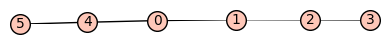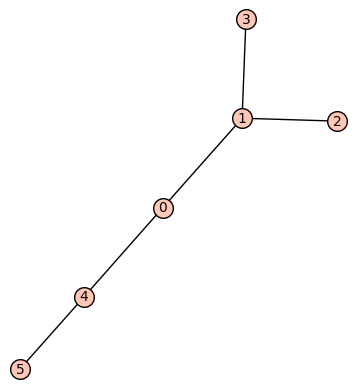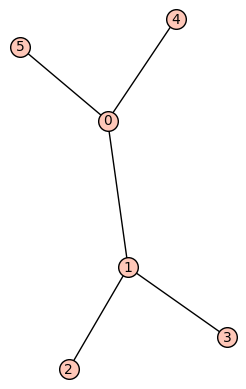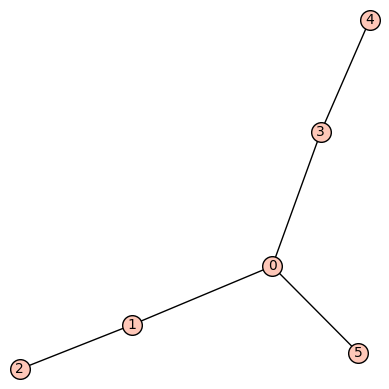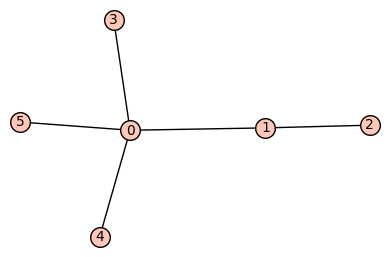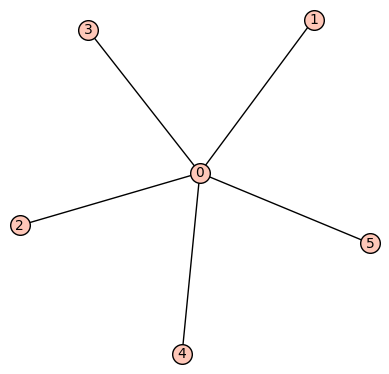2019-08-05 10:43:37 +0200 commented answer caterpillar graphs in sage Can you please explain to me what is the number of vertices and vertices of degree on in $G$? It keeps changing when I run. Thank you. 2019-08-05 08:57:15 +0200 asked a question caterpillar graphs in sage A caterpillar or caterpillar tree is a tree in which all the vertices are within distance 1 of a central path. How to define caterpillar graphs in sage? is it already implemented? Kindly help me with this. Thank you. 2019-08-04 08:32:11 +0200 commented answer graphs of order n Thank you. one doubt. graphs(5) gives the list of graphs up to isomorphism? 2019-08-03 15:13:02 +0200 asked a question graphs of order n How to generate all the graphs of particular order $n$ in sage? is it possible to do the same up to isomorphism at least for small $n$? Thank you. 2019-08-03 13:34:18 +0200 asked a question plot comment not working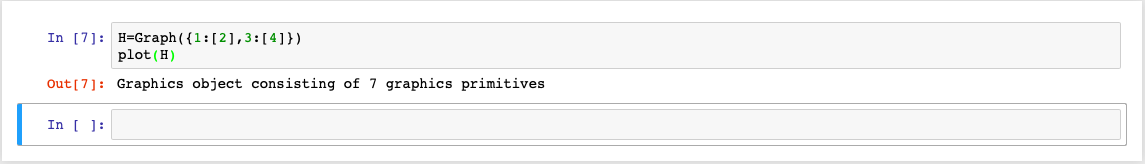Why the image of the graph is not displayed here? Kindly help me with resolving this. I am using Mac. Thank you. 2019-06-11 20:21:59 +0200 received badge ● Notable Question (source) 2019-01-19 16:39:09 +0200 asked a question periodicity of a word I have the following code to create words. sage: M. = FreeMonoid(3) sage: Word(x^3*y*x*z^2*x) word: xxxyxzzx  I want find the periodicity of a given word. For example ababab has periodicity 3 and abcdabcde has periodicity 1. Kindly help me with this. Thank you. 2018-10-10 09:04:43 +0200 asked a question Graph with partial edge labelling Hello all, I need to draw a graph in which some edges are labelled and some edges are not. I know how to make all the edges labelled. But I need mixed edges (some are labelled and some are not). Kindly help me with this. Thank you 2018-10-10 08:17:11 +0200 commented answer creating graph with dotted edges Also in the output graph with dotted edges and ordinary edges, I am getting axes also part of the output. But I need only the graph in order to add it in my paper. Kindly tell me how to get rid of this measurement axes. Thank yu. 2018-10-10 08:03:52 +0200 commented answer creating graph with dotted edges Thanks. It works nicely. Can you please tell me how to add labels only on certain edges? thank you. 2018-10-10 08:02:49 +0200 marked best answer creating graph with dotted edges Hello all, I need to draw a graph in which some edges are usual and some edges are dotted. I know how to make all the edges dotted (using the option "dotted" for the parameter edge_style of plot). But I need mixed edges (some are dotted and some are usual). Kindly help me with this. Thank you.